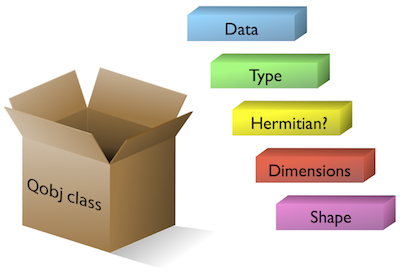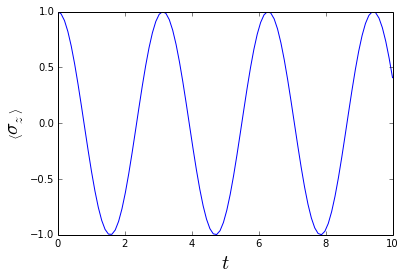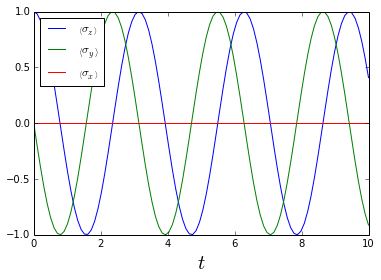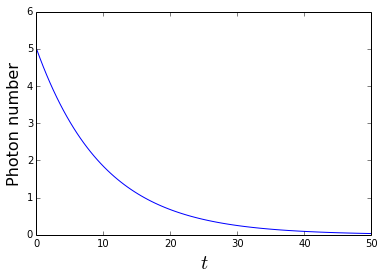Lecture 0 - Introduction to QuTiP - The Quantum Toolbox in Python¶

Author: J. R. Johansson ([email protected]), http://dml.riken.jp/~rob/

The latest version of this IPython notebook lecture is available at http://github.com/jrjohansson/qutip-lectures.

The other notebooks in this lecture series are indexed at http://jrjohansson.github.com.

In :
%matplotlib inline
import matplotlib.pyplot as plt
import numpy as np
from IPython.display import Image

Introduction¶

QuTiP is a python package for calculations and numerical simulations of quantum systems.

It includes facilities for representing and doing calculations with quantum objects such state vectors (wavefunctions), as bras/kets/density matrices, quantum operators of single and composite systems, and superoperators (useful for defining master equations).

It also includes solvers for a time-evolution of quantum systems, according to: Schrodinger equation, von Neuman equation, master equations, Floquet formalism, Monte-Carlo quantum trajectors, experimental implementations of the stochastic Schrodinger/master equations.

For more information see the project web site at http://qutip.googlecode.com, and the documentation at http://qutip.googlecode.com/svn/doc/2.1.0/html/index.html.

Installation¶

To install QuTiP, download the latest release from http://code.google.com/p/qutip/downloads/list or get the latest code from https://github.com/qutip/qutip, and run

$sudo python setup.py install in the source code directory. For more detailed installation instructions and a list of dependencies that must be installed on the system (basically python+cython+numpy+scipy+matplotlib), see http://qutip.googlecode.com/svn/doc/2.1.0/html/installation.html. To use QuTiP in a Python program, first inlude the qutip module: In : from qutip import * This will make the functions and classes in QuTiP available in the rest of the program. Quantum object class: qobj¶ At the heart of the QuTiP package is the Qobj class, which is used for representing quantum object such as states and operator. The Qobj class contains all the information required to describe a quantum system, such as its matrix representation, composite structure and dimensionality. In : Image(filename='images/qobj.png') Out:Creating and inspecting quantum objects¶ We can create a new quantum object using the Qobj class constructor, like this: In : q = Qobj([, ]) q Out:$\text{Quantum object: dims = [, ], shape = [2, 1], type = ket}\\[1em]\begin{pmatrix}1.0\\0.0\\\end{pmatrix}$Here we passed python list as an argument to the class constructor. The data in this list is used to construct the matrix representation of the quantum objects, and the other properties of the quantum object is by default computed from the same data. We can inspect the properties of a Qobj instance using the following class method: In : # the dimension, or composite Hilbert state space structure q.dims Out: [, ] In : # the shape of the matrix data representation q.shape Out: [2, 1] In : # the matrix data itself. in sparse matrix format. q.data Out: <2x1 sparse matrix of type '<type 'numpy.complex128'>' with 1 stored elements in Compressed Sparse Row format> In : # get the dense matrix representation q.full() Out: array([[ 1.+0.j], [ 0.+0.j]]) In : # some additional properties q.isherm, q.type Out: (False, 'ket') Using Qobj instances for calculations¶ With Qobj instances we can do arithmetic and apply a number of different operations using class methods: In : sy = Qobj([[0,-1j], [1j,0]]) # the sigma-y Pauli operator sy Out:$\text{Quantum object: dims = [, ], shape = [2, 2], type = oper, isherm = True}\\[1em]\begin{pmatrix}0.0 & -1.0j\\1.0j & 0.0\\\end{pmatrix}$In : sz = Qobj([[1,0], [0,-1]]) # the sigma-z Pauli operator sz Out:$\text{Quantum object: dims = [, ], shape = [2, 2], type = oper, isherm = True}\\[1em]\begin{pmatrix}1.0 & 0.0\\0.0 & -1.0\\\end{pmatrix}$In : # some arithmetic with quantum objects H = 1.0 * sz + 0.1 * sy print("Qubit Hamiltonian = \n") H Qubit Hamiltonian = Out:$\text{Quantum object: dims = [, ], shape = [2, 2], type = oper, isherm = True}\\[1em]\begin{pmatrix}1.0 & -0.100j\\0.100j & -1.0\\\end{pmatrix}$Example of modifying quantum objects using the Qobj methods: In : # The hermitian conjugate sy.dag() Out:$\text{Quantum object: dims = [, ], shape = [2, 2], type = oper, isherm = True}\\[1em]\begin{pmatrix}0.0 & -1.0j\\1.0j & 0.0\\\end{pmatrix}$In : # The trace H.tr() Out: 0.0 In : # Eigen energies H.eigenenergies() Out: array([-1.00498756, 1.00498756]) For a complete list of methods and properties of the Qobj class, see the QuTiP documentation or try help(Qobj) or dir(Qobj). States and operators¶ Normally we do not need to create Qobj instances from stratch, using its constructor and passing its matrix represantation as argument. Instead we can use functions in QuTiP that generates common states and operators for us. Here are some examples of built-in state functions: State vectors¶ In : # Fundamental basis states (Fock states of oscillator modes) N = 2 # number of states in the Hilbert space n = 1 # the state that will be occupied basis(N, n) # equivalent to fock(N, n) Out:$\text{Quantum object: dims = [, ], shape = [2, 1], type = ket}\\[1em]\begin{pmatrix}0.0\\1.0\\\end{pmatrix}$In : fock(4, 2) # another example Out:$\text{Quantum object: dims = [, ], shape = [4, 1], type = ket}\\[1em]\begin{pmatrix}0.0\\0.0\\1.0\\0.0\\\end{pmatrix}$In : # a coherent state coherent(N=10, alpha=1.0) Out:$\text{Quantum object: dims = [, ], shape = [10, 1], type = ket}\\[1em]\begin{pmatrix}0.607\\0.607\\0.429\\0.248\\0.124\\0.055\\0.023\\0.009\\0.003\\0.001\\\end{pmatrix}$Density matrices¶ In : # a fock state as density matrix fock_dm(5, 2) # 5 = hilbert space size, 2 = state that is occupied Out:$\text{Quantum object: dims = [, ], shape = [5, 5], type = oper, isherm = True}\\[1em]\begin{pmatrix}0.0 & 0.0 & 0.0 & 0.0 & 0.0\\0.0 & 0.0 & 0.0 & 0.0 & 0.0\\0.0 & 0.0 & 1.0 & 0.0 & 0.0\\0.0 & 0.0 & 0.0 & 0.0 & 0.0\\0.0 & 0.0 & 0.0 & 0.0 & 0.0\\\end{pmatrix}$In : # coherent state as density matrix coherent_dm(N=8, alpha=1.0) Out:$\text{Quantum object: dims = [, ], shape = [8, 8], type = oper, isherm = True}\\[1em]\begin{pmatrix}0.368 & 0.368 & 0.260 & 0.150 & 0.075 & 0.034 & 0.014 & 0.006\\0.368 & 0.368 & 0.260 & 0.150 & 0.075 & 0.034 & 0.014 & 0.006\\0.260 & 0.260 & 0.184 & 0.106 & 0.053 & 0.024 & 0.010 & 0.004\\0.150 & 0.150 & 0.106 & 0.061 & 0.031 & 0.014 & 0.006 & 0.002\\0.075 & 0.075 & 0.053 & 0.031 & 0.015 & 0.007 & 0.003 & 0.001\\0.034 & 0.034 & 0.024 & 0.014 & 0.007 & 0.003 & 0.001 & 5.276\times10^{-04}\\0.014 & 0.014 & 0.010 & 0.006 & 0.003 & 0.001 & 4.990\times10^{-04} & 2.126\times10^{-04}\\0.006 & 0.006 & 0.004 & 0.002 & 0.001 & 5.276\times10^{-04} & 2.126\times10^{-04} & 9.058\times10^{-05}\\\end{pmatrix}$In : # thermal state n = 1 # average number of thermal photons thermal_dm(8, n) Out:$\text{Quantum object: dims = [, ], shape = [8, 8], type = oper, isherm = True}\\[1em]\begin{pmatrix}0.502 & 0.0 & 0.0 & 0.0 & 0.0 & 0.0 & 0.0 & 0.0\\0.0 & 0.251 & 0.0 & 0.0 & 0.0 & 0.0 & 0.0 & 0.0\\0.0 & 0.0 & 0.125 & 0.0 & 0.0 & 0.0 & 0.0 & 0.0\\0.0 & 0.0 & 0.0 & 0.063 & 0.0 & 0.0 & 0.0 & 0.0\\0.0 & 0.0 & 0.0 & 0.0 & 0.031 & 0.0 & 0.0 & 0.0\\0.0 & 0.0 & 0.0 & 0.0 & 0.0 & 0.016 & 0.0 & 0.0\\0.0 & 0.0 & 0.0 & 0.0 & 0.0 & 0.0 & 0.008 & 0.0\\0.0 & 0.0 & 0.0 & 0.0 & 0.0 & 0.0 & 0.0 & 0.004\\\end{pmatrix}$Operators¶ Qubit (two-level system) operators¶ In : # Pauli sigma x sigmax() Out:$\text{Quantum object: dims = [, ], shape = [2, 2], type = oper, isherm = True}\\[1em]\begin{pmatrix}0.0 & 1.0\\1.0 & 0.0\\\end{pmatrix}$In : # Pauli sigma y sigmay() Out:$\text{Quantum object: dims = [, ], shape = [2, 2], type = oper, isherm = True}\\[1em]\begin{pmatrix}0.0 & -1.0j\\1.0j & 0.0\\\end{pmatrix}$In : # Pauli sigma z sigmaz() Out:$\text{Quantum object: dims = [, ], shape = [2, 2], type = oper, isherm = True}\\[1em]\begin{pmatrix}1.0 & 0.0\\0.0 & -1.0\\\end{pmatrix}$Harmonic oscillator operators¶ In : # annihilation operator destroy(N=8) # N = number of fock states included in the Hilbert space Out:$\text{Quantum object: dims = [, ], shape = [8, 8], type = oper, isherm = False}\\[1em]\begin{pmatrix}0.0 & 1.0 & 0.0 & 0.0 & 0.0 & 0.0 & 0.0 & 0.0\\0.0 & 0.0 & 1.414 & 0.0 & 0.0 & 0.0 & 0.0 & 0.0\\0.0 & 0.0 & 0.0 & 1.732 & 0.0 & 0.0 & 0.0 & 0.0\\0.0 & 0.0 & 0.0 & 0.0 & 2.0 & 0.0 & 0.0 & 0.0\\0.0 & 0.0 & 0.0 & 0.0 & 0.0 & 2.236 & 0.0 & 0.0\\0.0 & 0.0 & 0.0 & 0.0 & 0.0 & 0.0 & 2.449 & 0.0\\0.0 & 0.0 & 0.0 & 0.0 & 0.0 & 0.0 & 0.0 & 2.646\\0.0 & 0.0 & 0.0 & 0.0 & 0.0 & 0.0 & 0.0 & 0.0\\\end{pmatrix}$In : # creation operator create(N=8) # equivalent to destroy(5).dag() Out:$\text{Quantum object: dims = [, ], shape = [8, 8], type = oper, isherm = False}\\[1em]\begin{pmatrix}0.0 & 0.0 & 0.0 & 0.0 & 0.0 & 0.0 & 0.0 & 0.0\\1.0 & 0.0 & 0.0 & 0.0 & 0.0 & 0.0 & 0.0 & 0.0\\0.0 & 1.414 & 0.0 & 0.0 & 0.0 & 0.0 & 0.0 & 0.0\\0.0 & 0.0 & 1.732 & 0.0 & 0.0 & 0.0 & 0.0 & 0.0\\0.0 & 0.0 & 0.0 & 2.0 & 0.0 & 0.0 & 0.0 & 0.0\\0.0 & 0.0 & 0.0 & 0.0 & 2.236 & 0.0 & 0.0 & 0.0\\0.0 & 0.0 & 0.0 & 0.0 & 0.0 & 2.449 & 0.0 & 0.0\\0.0 & 0.0 & 0.0 & 0.0 & 0.0 & 0.0 & 2.646 & 0.0\\\end{pmatrix}$In : # the position operator is easily constructed from the annihilation operator a = destroy(8) x = a + a.dag() x Out:$\text{Quantum object: dims = [, ], shape = [8, 8], type = oper, isherm = True}\\[1em]\begin{pmatrix}0.0 & 1.0 & 0.0 & 0.0 & 0.0 & 0.0 & 0.0 & 0.0\\1.0 & 0.0 & 1.414 & 0.0 & 0.0 & 0.0 & 0.0 & 0.0\\0.0 & 1.414 & 0.0 & 1.732 & 0.0 & 0.0 & 0.0 & 0.0\\0.0 & 0.0 & 1.732 & 0.0 & 2.0 & 0.0 & 0.0 & 0.0\\0.0 & 0.0 & 0.0 & 2.0 & 0.0 & 2.236 & 0.0 & 0.0\\0.0 & 0.0 & 0.0 & 0.0 & 2.236 & 0.0 & 2.449 & 0.0\\0.0 & 0.0 & 0.0 & 0.0 & 0.0 & 2.449 & 0.0 & 2.646\\0.0 & 0.0 & 0.0 & 0.0 & 0.0 & 0.0 & 2.646 & 0.0\\\end{pmatrix}$Using Qobj instances we can check some well known commutation relations:¶ In : def commutator(op1, op2): return op1 * op2 - op2 * op1$[a, a^1] = 1$In : a = destroy(5) commutator(a, a.dag()) Out:$\text{Quantum object: dims = [, ], shape = [5, 5], type = oper, isherm = True}\\[1em]\begin{pmatrix}1.0 & 0.0 & 0.0 & 0.0 & 0.0\\0.0 & 1.0 & 0.0 & 0.0 & 0.0\\0.0 & 0.0 & 1.000 & 0.0 & 0.0\\0.0 & 0.0 & 0.0 & 1.0 & 0.0\\0.0 & 0.0 & 0.0 & 0.0 & -4.0\\\end{pmatrix}$Ops... The result is not identity! Why? Because we have truncated the Hilbert space. But that's OK as long as the highest Fock state isn't involved in the dynamics in our truncated Hilbert space. If it is, the approximation that the truncation introduces might be a problem.$[x,p] = i$In : x = (a + a.dag())/sqrt(2) p = -1j * (a - a.dag())/sqrt(2) In : commutator(x, p) Out:$\text{Quantum object: dims = [, ], shape = [5, 5], type = oper, isherm = False}\\[1em]\begin{pmatrix}1.000j & 0.0 & 0.0 & 0.0 & 0.0\\0.0 & 1.0j & 0.0 & 0.0 & 0.0\\0.0 & 0.0 & 1.000j & 0.0 & 0.0\\0.0 & 0.0 & 0.0 & 1.000j & 0.0\\0.0 & 0.0 & 0.0 & 0.0 & -4.000j\\\end{pmatrix}$Same issue with the truncated Hilbert space, but otherwise OK. Let's try some Pauli spin inequalities$[\sigma_x, \sigma_y] = 2i \sigma_z$In : commutator(sigmax(), sigmay()) - 2j * sigmaz() Out:$\text{Quantum object: dims = [, ], shape = [2, 2], type = oper, isherm = True}\\[1em]\begin{pmatrix}0.0 & 0.0\\0.0 & 0.0\\\end{pmatrix}-i \sigma_x \sigma_y \sigma_z = \mathbf{1}$In : -1j * sigmax() * sigmay() * sigmaz() Out:$\text{Quantum object: dims = [, ], shape = [2, 2], type = oper, isherm = True}\\[1em]\begin{pmatrix}1.0 & 0.0\\0.0 & 1.0\\\end{pmatrix}\sigma_x^2 = \sigma_y^2 = \sigma_z^2 = \mathbf{1}$In : sigmax()**2 == sigmay()**2 == sigmaz()**2 == qeye(2) Out: True Composite systems¶ In most cases we are interested in coupled quantum systems, for example coupled qubits, a qubit coupled to a cavity (oscillator mode), etc. To define states and operators for such systems in QuTiP, we use the tensor function to create Qobj instances for the composite system. For example, consider a system composed of two qubits. If we want to create a Pauli$\sigma_z$operator that acts on the first qubit and leaves the second qubit unaffected (i.e., the operator$\sigma_z \otimes \mathbf{1}$), we would do: In : sz1 = tensor(sigmaz(), qeye(2)) sz1 Out:$\text{Quantum object: dims = [[2, 2], [2, 2]], shape = [4, 4], type = oper, isherm = True}\\[1em]\begin{pmatrix}1.0 & 0.0 & 0.0 & 0.0\\0.0 & 1.0 & 0.0 & 0.0\\0.0 & 0.0 & -1.0 & 0.0\\0.0 & 0.0 & 0.0 & -1.0\\\end{pmatrix}$We can easily verify that this two-qubit operator does indeed have the desired properties: In : psi1 = tensor(basis(N,1), basis(N,0)) # excited first qubit psi2 = tensor(basis(N,0), basis(N,1)) # excited second qubit In : sz1 * psi1 == psi1 # this should not be true, because sz1 should flip the sign of the excited state of psi1 Out: False In : sz1 * psi2 == psi2 # this should be true, because sz1 should leave psi2 unaffected Out: True Above we used the qeye(N) function, which generates the identity operator with N quantum states. If we want to do the same thing for the second qubit we can do: In : sz2 = tensor(qeye(2), sigmaz()) sz2 Out:$\text{Quantum object: dims = [[2, 2], [2, 2]], shape = [4, 4], type = oper, isherm = True}\\[1em]\begin{pmatrix}1.0 & 0.0 & 0.0 & 0.0\\0.0 & -1.0 & 0.0 & 0.0\\0.0 & 0.0 & 1.0 & 0.0\\0.0 & 0.0 & 0.0 & -1.0\\\end{pmatrix}$Note the order of the argument to the tensor function, and the correspondingly different matrix representation of the two operators sz1 and sz2. Using the same method we can create coupling terms of the form$\sigma_x \otimes \sigma_x$: In : tensor(sigmax(), sigmax()) Out:$\text{Quantum object: dims = [[2, 2], [2, 2]], shape = [4, 4], type = oper, isherm = True}\\[1em]\begin{pmatrix}0.0 & 0.0 & 0.0 & 1.0\\0.0 & 0.0 & 1.0 & 0.0\\0.0 & 1.0 & 0.0 & 0.0\\1.0 & 0.0 & 0.0 & 0.0\\\end{pmatrix}$Now we are ready to create a Qobj representation of a coupled two-qubit Hamiltonian:$H = \epsilon_1 \sigma_z^{(1)} + \epsilon_2 \sigma_z^{(2)} + g \sigma_x^{(1)}\sigma_x^{(2)}$In : epsilon = [1.0, 1.0] g = 0.1 sz1 = tensor(sigmaz(), qeye(2)) sz2 = tensor(qeye(2), sigmaz()) H = epsilon * sz1 + epsilon * sz2 + g * tensor(sigmax(), sigmax()) H Out:$\text{Quantum object: dims = [[2, 2], [2, 2]], shape = [4, 4], type = oper, isherm = True}\\[1em]\begin{pmatrix}2.0 & 0.0 & 0.0 & 0.100\\0.0 & 0.0 & 0.100 & 0.0\\0.0 & 0.100 & 0.0 & 0.0\\0.100 & 0.0 & 0.0 & -2.0\\\end{pmatrix}$To create composite systems of different types, all we need to do is to change the operators that we pass to the tensor function (which can take an arbitrary number of operator for composite systems with many components). For example, the Jaynes-Cumming Hamiltonian for a qubit-cavity system:$H = \omega_c a^\dagger a - \frac{1}{2}\omega_a \sigma_z + g (a \sigma_+ + a^\dagger \sigma_-)$In : wc = 1.0 # cavity frequency wa = 1.0 # qubit/atom frenqency g = 0.1 # coupling strength # cavity mode operator a = tensor(destroy(5), qeye(2)) # qubit/atom operators sz = tensor(qeye(5), sigmaz()) # sigma-z operator sm = tensor(qeye(5), destroy(2)) # sigma-minus operator # the Jaynes-Cumming Hamiltonian H = wc * a.dag() * a - 0.5 * wa * sz + g * (a * sm.dag() + a.dag() * sm) H Out:$\text{Quantum object: dims = [[5, 2], [5, 2]], shape = [10, 10], type = oper, isherm = True}\\[1em]\begin{pmatrix}-0.500 & 0.0 & 0.0 & 0.0 & 0.0 & 0.0 & 0.0 & 0.0 & 0.0 & 0.0\\0.0 & 0.500 & 0.100 & 0.0 & 0.0 & 0.0 & 0.0 & 0.0 & 0.0 & 0.0\\0.0 & 0.100 & 0.500 & 0.0 & 0.0 & 0.0 & 0.0 & 0.0 & 0.0 & 0.0\\0.0 & 0.0 & 0.0 & 1.500 & 0.141 & 0.0 & 0.0 & 0.0 & 0.0 & 0.0\\0.0 & 0.0 & 0.0 & 0.141 & 1.500 & 0.0 & 0.0 & 0.0 & 0.0 & 0.0\\0.0 & 0.0 & 0.0 & 0.0 & 0.0 & 2.500 & 0.173 & 0.0 & 0.0 & 0.0\\0.0 & 0.0 & 0.0 & 0.0 & 0.0 & 0.173 & 2.500 & 0.0 & 0.0 & 0.0\\0.0 & 0.0 & 0.0 & 0.0 & 0.0 & 0.0 & 0.0 & 3.500 & 0.200 & 0.0\\0.0 & 0.0 & 0.0 & 0.0 & 0.0 & 0.0 & 0.0 & 0.200 & 3.500 & 0.0\\0.0 & 0.0 & 0.0 & 0.0 & 0.0 & 0.0 & 0.0 & 0.0 & 0.0 & 4.500\\\end{pmatrix}$Note that$a \sigma_+ = (a \otimes \mathbf{1}) (\mathbf{1} \otimes \sigma_+)$so the following two are identical: In : a = tensor(destroy(3), qeye(2)) sp = tensor(qeye(3), create(2)) a * sp Out:$\text{Quantum object: dims = [[3, 2], [3, 2]], shape = [6, 6], type = oper, isherm = False}\\[1em]\begin{pmatrix}0.0 & 0.0 & 0.0 & 0.0 & 0.0 & 0.0\\0.0 & 0.0 & 1.0 & 0.0 & 0.0 & 0.0\\0.0 & 0.0 & 0.0 & 0.0 & 0.0 & 0.0\\0.0 & 0.0 & 0.0 & 0.0 & 1.414 & 0.0\\0.0 & 0.0 & 0.0 & 0.0 & 0.0 & 0.0\\0.0 & 0.0 & 0.0 & 0.0 & 0.0 & 0.0\\\end{pmatrix}$In : tensor(destroy(3), create(2)) Out:$\text{Quantum object: dims = [[3, 2], [3, 2]], shape = [6, 6], type = oper, isherm = False}\\[1em]\begin{pmatrix}0.0 & 0.0 & 0.0 & 0.0 & 0.0 & 0.0\\0.0 & 0.0 & 1.0 & 0.0 & 0.0 & 0.0\\0.0 & 0.0 & 0.0 & 0.0 & 0.0 & 0.0\\0.0 & 0.0 & 0.0 & 0.0 & 1.414 & 0.0\\0.0 & 0.0 & 0.0 & 0.0 & 0.0 & 0.0\\0.0 & 0.0 & 0.0 & 0.0 & 0.0 & 0.0\\\end{pmatrix}$Unitary dynamics¶ Unitary evolution of a quantum system in QuTiP can be calculated with the mesolve function. mesolve is short for Master-eqaution solve (for dissipative dynamics), but if no collapse operators (which describe the dissipation) are given to the solve it falls back on the unitary evolution of the Schrodinger (for initial states in state vector for) or the von Neuman equation (for initial states in density matrix form). The evolution solvers in QuTiP returns a class of type Odedata, which contains the solution to the problem posed to the evolution solver. For example, considor a qubit with Hamiltonian$H = \sigma_x$and initial state$\left|1\right>$(in the sigma-z basis): Its evolution can be calculated as follows: In : # Hamiltonian H = sigmax() # initial state psi0 = basis(2, 0) # list of times for which the solver should store the state vector tlist = np.linspace(0, 10, 100) result = mesolve(H, psi0, tlist, [], []) In : result Out: Odedata object with sesolve data. --------------------------------- states = True num_collapse = 0 The result object contains a list of the wavefunctions at the times requested with the tlist array. In : len(result.states) Out: 100 In : result.states[-1] # the finial state Out:$\text{Quantum object: dims = [, ], shape = [2, 1], type = ket}\\[1em]\begin{pmatrix}-0.839\\0.544j\\\end{pmatrix}$Expectation values¶ The expectation values of an operator given a state vector or density matrix (or list thereof) can be calculated using the expect function. In : expect(sigmaz(), result.states[-1]) Out: 0.40810176186454994 In : expect(sigmaz(), result.states) Out: array([ 1. , 0.97966324, 0.91948013, 0.82189857, 0.69088756, 0.53177579, 0.3510349 , 0.15601625, -0.04534808, -0.24486795, -0.43442821, -0.60631884, -0.75354841, -0.87012859, -0.95131766, -0.99381332, -0.99588712, -0.95745468, -0.88007921, -0.76690787, -0.62254375, -0.45285867, -0.26475429, -0.06588149, 0.13567091, 0.33170513, 0.51424779, 0.67587427, 0.81001063, 0.91120109, 0.97532984, 0.99978853, 0.9835823 , 0.92737033, 0.83343897, 0.70560878, 0.54907906, 0.37021643, 0.17629587, -0.02479521, -0.22487778, -0.41581382, -0.58983733, -0.73987014, -0.85980992, -0.94477826, -0.9913192 , -0.99753971, -0.96318677, -0.88965766, -0.77994308, -0.63850553, -0.4710978 , -0.28452892, -0.08638732, 0.11526793, 0.31223484, 0.49650212, 0.660575 , 0.79778003, 0.90253662, 0.97058393, 0.99915421, 0.98708537, 0.9348683 , 0.84462688, 0.72003156, 0.56615011, 0.38924141, 0.19650096, -0.00423183, -0.20479249, -0.39702355, -0.57310633, -0.72587894, -0.84912758, -0.93783928, -0.9884058 , -0.99877041, -0.9685115 , -0.89885984, -0.79264843, -0.65419728, -0.4891377 , -0.30418323, -0.10685663, 0.09481617, 0.29263248, 0.47854644, 0.64499632, 0.785212 , 0.89349043, 0.96542751, 0.9980973 , 0.99017096, 0.94197089, 0.85545757, 0.73414984, 0.58298172, 0.40810176]) In : fig, axes = plt.subplots(1,1) axes.plot(tlist, expect(sigmaz(), result.states)) axes.set_xlabel(r'$t$', fontsize=20) axes.set_ylabel(r'$\left<\sigma_z\right>$', fontsize=20);If we are only interested in expectation values, we could pass a list of operators to the mesolve function that we want expectation values for, and have the solver compute then and store the results in the Odedata class instance that it returns. For example, to request that the solver calculates the expectation values for the operators$\sigma_x, \sigma_y, \sigma_z$: In : result = mesolve(H, psi0, tlist, [], [sigmax(), sigmay(), sigmaz()]) Now the expectation values are available in result.expect, result.expect, and result.expect: In : fig, axes = plt.subplots(1,1) axes.plot(tlist, result.expect, label=r'$\left<\sigma_z\right>$') axes.plot(tlist, result.expect, label=r'$\left<\sigma_y\right>$') axes.plot(tlist, result.expect, label=r'$\left<\sigma_x\right>$') axes.set_xlabel(r'$t$', fontsize=20) axes.legend(loc=2);Dissipative dynamics¶ To add dissipation to a problem, all we need to do is to define a list of collapse operators to the call to the mesolve solver. A collapse operator is an operator that describes how the system is interacting with its environment. For example, consider a quantum harmonic oscillator with Hamiltonian$H = \hbar\omega a^\dagger a$and which loses photons to its environment with a relaxation rate$\kappa$. The collapse operator that describes this process is$\sqrt{\kappa} a$since$a$is the photon annihilation operator of the oscillator. To program this problem in QuTiP: In : w = 1.0 # oscillator frequency kappa = 0.1 # relaxation rate a = destroy(10) # oscillator annihilation operator rho0 = fock_dm(10, 5) # initial state, fock state with 5 photons H = w * a.dag() * a # Hamiltonian # A list of collapse operators c_ops = [sqrt(kappa) * a] In : tlist = np.linspace(0, 50, 100) # request that the solver return the expectation value of the photon number state operator a.dag() * a result = mesolve(H, rho0, tlist, c_ops, [a.dag() * a]) In : fig, axes = plt.subplots(1,1) axes.plot(tlist, result.expect) axes.set_xlabel(r'$t\$', fontsize=20)
axes.set_ylabel(r"Photon number", fontsize=16);Software versions¶

In :
from qutip.ipynbtools import version_table

version_table()
Out:
SoftwareVersion
Cython0.20.1
SciPy0.13.3
QuTiP3.0.0.dev-927c867
Python2.7.5+ (default, Feb 27 2014, 19:37:08) [GCC 4.8.1]
IPython2.0.0
OSposix [linux2]
Numpy1.8.1
matplotlib1.3.1
Wed Jul 02 15:30:51 2014 JST